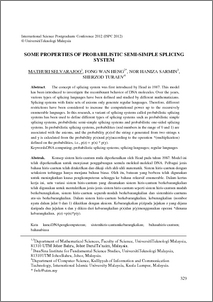# Some properties of probabilistic semi-simple splicing systems

Mathuri, S. and Fong, Wan Heng and Sarmin, Nor Haniza and Turaev, Sherzod (2012) Some properties of probabilistic semi-simple splicing systems. In: International Science Postgraduate Conference 2012 (ISPC 2012), 27-30 November 2012, Skudai, Johor.Preview
PDF (Some properties of probabilistic semi-simple splicing systems) - Published Version

## Abstract

The concept of splicing system was first introduced by Head in 1987. This model has been introduced to investigate the recombinant behavior of DNA molecules. Over the years, various types of splicing languages have been defined and studied by different mathematicians. Splicing systems with finite sets of axioms only generate regular languages. Therefore, different restrictions have been considered to increase the computational power up to the recursively enumerable languages. In this research, a variant of splicing systems called probabilistic splicing systems has been used to define different types of splicing systems such as probabilistic simple splicing systems, probabilistic semi-simple splicing systems and probabilistic one-sided splicing systems. In probabilistic splicing systems, probabilities (real numbers in the range of 0 and 1) are associated with the axioms, and the probability p(z)of the string z generated from two strings x and y is calculated from the probability p(x)and p(y) according to the operation *(multiplication) defined on the probabilities, i.e., p(z) = p(x) * p(y).

Item Type: Conference or Workshop Item (Full Paper) DNA computing; probabilistic splicing systems; splicing languages; regular languages Q Science > QA MathematicsQ Science > QA Mathematics > QA75 Electronic computers. Computer science Kulliyyah of Information and Communication Technology > Department of Computer ScienceKulliyyah of Information and Communication Technology > Department of Computer Science Dr. Sherzod Turaev 27 Dec 2012 10:31 27 Dec 2012 10:31 http://irep.iium.edu.my/id/eprint/27319View Item